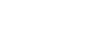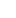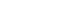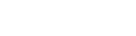xDoubling timeEncyclopedia
The doubling time is the period of time required for a quantity to double in size or value. It is applied to population growth
Population growth
Population growth is the change in a population over time, and can be quantified as the change in the number of individuals of any species in a population using "per unit time" for measurement....

, inflation
Inflation
In economics, inflation is a rise in the general level of prices of goods and services in an economy over a period of time.When the general price level rises, each unit of currency buys fewer goods and services. Consequently, inflation also reflects an erosion in the purchasing power of money – a...

, resource extraction
Resource extraction
The related terms natural resource extraction both refer to the practice of locating, acquiring and selling natural resources....

, consumption
Consumption (economics)
Consumption is a common concept in economics, and gives rise to derived concepts such as consumer debt. Generally, consumption is defined in part by comparison to production. But the precise definition can vary because different schools of economists define production quite differently...

of goods, compound interest
Compound interest
Compound interest arises when interest is added to the principal, so that from that moment on, the interest that has been added also itself earns interest. This addition of interest to the principal is called compounding...

, the volume of malignant tumours
Cancer
Cancer , known medically as a malignant neoplasm, is a large group of different diseases, all involving unregulated cell growth. In cancer, cells divide and grow uncontrollably, forming malignant tumors, and invade nearby parts of the body. The cancer may also spread to more distant parts of the...

, and many other things which tend to grow over time. When the relative growth rate (not the absolute growth rate) is constant, the quantity undergoes exponential growth
Exponential growth
Exponential growth occurs when the growth rate of a mathematical function is proportional to the function's current value...

and has a constant doubling time or period which can be calculated directly from the growth rate.

This time can be calculated by dividing the natural logarithm
Natural logarithm
The natural logarithm is the logarithm to the base e, where e is an irrational and transcendental constant approximately equal to 2.718281828...

of 2 by the exponent of growth, or approximated by dividing 70 by the percentage growth rate (more roughly but roundly, dividing 72; see the rule of 72
Rule of 72
In finance, the rule of 72, the rule of 70 and the rule of 69 are methods for estimating an investment's doubling time. The rule number is divided by the interest percentage per period to obtain the approximate number of periods required for doubling...

for details and a derivation of this formula).

The doubling time is a characteristic unit (a natural unit of scale) for the exponential growth equation, and its converse for exponential decay is the half-life
Half-life
Half-life, abbreviated t½, is the period of time it takes for the amount of a substance undergoing decay to decrease by half. The name was originally used to describe a characteristic of unstable atoms , but it may apply to any quantity which follows a set-rate decay.The original term, dating to...

.

For example, given Canada's net population growth of 0.9% in the year 2006, dividing 70 by 0.9 gives an approximate doubling time of 78 years. Thus if the growth rate remains constant, Canada's population would double from its 2006 figure of 33 million to 66 million by 2084.

## History

The notion of doubling time dates to interest on loans in Babylonian mathematics
Babylonian mathematics
Babylonian mathematics refers to any mathematics of the people of Mesopotamia, from the days of the early Sumerians to the fall of Babylon in 539 BC. Babylonian mathematical texts are plentiful and well edited...

. Clay tablets from circa 2000 BCE include the exercise "Given an interest rate of 1/60 per month (no compounding), come the doubling time." This yields an annual interest rate of 12/60 = 20%, and hence a doubling time of 100% growth/20% growth per year = 5 years.

Further, repaying double the initial amount of a loan, after a fixed time, was common commercial practice of the period: a common Assyrian loan of 1900 BCE consisted of loaning 2 minas of gold, getting back 4 in five years, and an Egyptian proverb of the time was "If wealth is placed where it bears interest, it comes back to you redoubled."

## Examination

Examining the doubling time can give a more intuitive sense of the long-term impact of growth than simply viewing the percentage growth rate.

For a constant growth rate of r%, the formula for the doubling time Td is given bySome doubling times calculated with this formula are shown in this table.

Simple doubling time formula:• N(t) = the number of objects at time t
• d = doubling period (time it takes for object to double in number)
• c= initial number of objects
• t = time

Doubling times Td given constant r% growth

r% Td
0.1 693.49
0.2 346.92
0.3 231.40
0.4 173.63
0.5 138.98
0.6 115.87
0.7 99.36
0.8 86.99
0.9 77.36
1.0 69.66

r% Td
1.1 63.36
1.2 58.11
1.3 53.66
1.4 49.86
1.5 46.56
1.6 43.67
1.7 41.12
1.8 38.85
1.9 36.83
2.0 35.00

r% Td
2.1 33.35
2.2 31.85
2.3 30.48
2.4 29.23
2.5 28.07
2.6 27.00
2.7 26.02
2.8 25.10
2.9 24.25
3.0 23.45

r% Td
3.1 22.70
3.2 22.01
3.3 21.35
3.4 20.73
3.5 20.15
3.6 19.60
3.7 19.08
3.8 18.59
3.9 18.12
4.0 17.67

r% Td
4.1 17.25
4.2 16.85
4.3 16.46
4.4 16.10
4.5 15.75
4.6 15.41
4.7 15.09
4.8 14.78
4.9 14.49
5.0 14.21

r% Td
5.5 12.95
6.0 11.90
6.5 11.01
7.0 10.24
7.5 9.58
8.0 9.01
8.5 8.50
9.0 8.04
9.5 7.64
10.0 7.27

r% Td
11.0 6.64
12.0 6.12
13.0 5.67
14.0 5.29
15.0 4.96
16.0 4.67
17.0 4.41
18.0 4.19
19.0 3.98
20.0 3.80

For example with an annual growth rate of 4.8% the doubling time is 14.78 years, and a doubling time of 10 years corresponds to a growth rate between 7% and 7.5% (actually about 7.18%).

When applied to the constant growth in consumption of a resource, the total amount consumed in one doubling period equals the total amount consumed in all previous periods. This enabled US President Jimmy Carter to note in a speech in 1977 that in each of the previous two decades the world had used more oil than in all of previous history, because of the roughly exponential growth in world oil consumption between 1950 and 1970 with a doubling period of under a decade.

Given two measurements of a growing quantity, q1 at time t1 and q2 at time t2, and assuming a constant growth rate, you can calculate the doubling time as## Where is it useful?

A constant relative growth rate means simply that the increase per unit time is proportional to the current quantity, i.e. the addition rate per unit amount is constant. It naturally occurs when the existing material generates or is the main determinant of new material. For example population growth in virgin territory, or fractional-reserve banking
Fractional-reserve banking
Fractional-reserve banking is a form of banking where banks maintain reserves that are only a fraction of the customer's deposits. Funds deposited into a bank are mostly lent out, and a bank keeps only a fraction of the quantity of deposits as reserves...

creating inflation. With unvarying growth the doubling calculation may be applied for many doubling periods or generations.

In practice eventually other constraints become important, exponential growth stops and the doubling time changes or becomes inapplicable. Limited food supply or other resources at high population densities will reduce growth, or needing a wheel-barrow full of notes to buy a loaf of bread will reduce the acceptance of paper money. While using doubling times is convenient and simple, we should not apply the idea without considering factors which may affect future growth. In the 1950s Canada's population growth rate was over 3% per year, so extrapolating the current growth rate of 0.9% for many decades (implied by the doubling time) is unjustified unless we have examined the underlying causes of the growth and determined they will not be changing significantly over that period.

The equivalent concept to doubling time for a material undergoing a constant negative relative growth rate or exponential decay is the half-life
Half-life
Half-life, abbreviated t½, is the period of time it takes for the amount of a substance undergoing decay to decrease by half. The name was originally used to describe a characteristic of unstable atoms , but it may apply to any quantity which follows a set-rate decay.The original term, dating to...

.

## Difference with generation time

Though in some contexts the two expressions are used interchangeably, in cell biology there is an often misunderstood difference. The generation time is the time between two cell divisions, which is only the same as the doubling time for the whole population when negative feedback factors are not significant, e.g. limited resources, suboptimal environmental conditions, or accumulation of waste concentrations. In these cases, a logistic growth model may apply.

However, in most cases, doubling time and generation time are the same.

## External links

The source of this article is wikipedia, the free encyclopedia.  The text of this article is licensed under the GFDL.